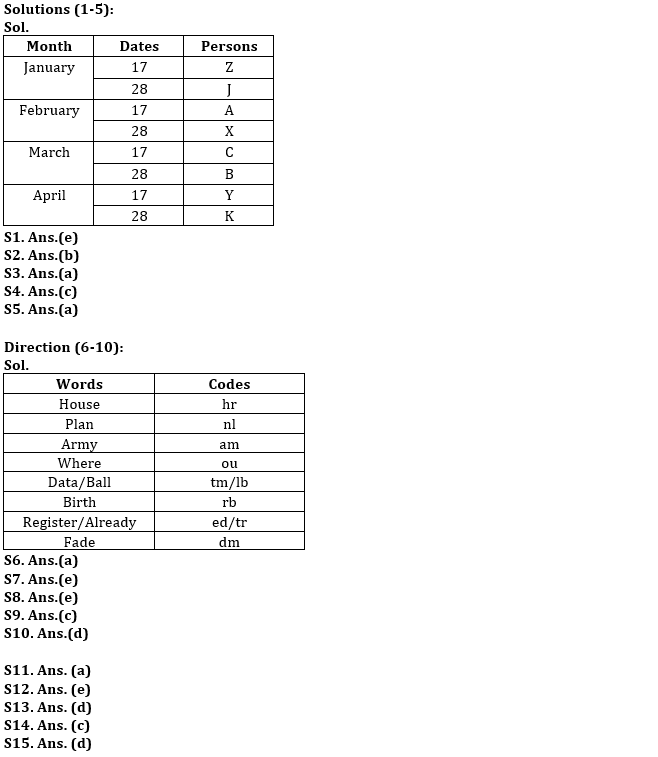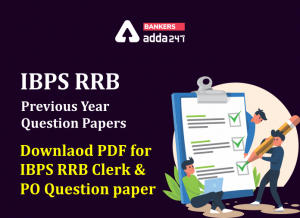Latest Banking jobs   »

# Reasoning Ability Quiz For IBPS RRB PO Clerk Prelims 2022- 23rd July

Directions (1-5): Study the information carefully and answer the questions given below.
Eight persons X, Y, Z, A, B, C, J and K attend lectures on two different dates 17th and 28th of four different months viz. January, February, March and April of the same year.
J attend lecture on an even date before X. Two persons attend lecture between X and Y. Z attends lecture before C. K attends lecture after Y. X attends lecture in the month which has least number of days. Three persons attend lecture between Z and C. Z does not attends lecture on an even number date. B does not attend lecture in the month having least number of days. Y does not attend lecture before B.

Q1. How many persons attends lecture between X and C?
(a) One
(b) Two
(c) Three
(d) Four
(e) None

Q2. Who among the following attends lecture on 28th March?
(a) Y
(b) B
(c) K
(d) X
(e) Z

Q3. Who among the following attends lecture immediately after X?
(a) C
(b) A
(c) B
(d) Z
(e) None of these

Q4. Four among the following five belongs to a group. Who does not belong to the group?
(a) J
(b) X
(c) A
(d) B
(e) K

Q5. Who among the following attends lecture on 17th of April?
(a) Y
(b) K
(c) Z
(d) A
(e) B

Directions (6-10): Study the following information carefully and answer the questions given below:
In a certain code language
‘Where house plan army’ is written as ‘nl am hr ou’,
‘Ball house birth data’ is written as ‘tm rb lb hr’,
‘Army plan register already’ is written as ‘ed nl am tr’,
‘Fade plan data ball’ is written as ‘tm lb nl dm’.

Q6. What is the code for ‘Fade’?
(a) dm
(b) nl
(c) lb
(d) tm
(e) None of these

Q7. Which of the following may be the code for ‘already house plan’?
(a) ed hr rb
(b) tr nl hr
(c) rb hr tr
(d) ed nl hr
(e) Either (b) or (d)

Q8. What is the code for ‘Register’?
(a) tr
(b) am
(c) nl
(d) ed
(e) Can’t be determined

Q9. What does ‘rb’ stand for?
(a) House
(b) Ball
(c) Birth
(d) Data
(e) None of these

Q10. What is the code for ‘Plan’?
(a) am
(b) hr
(c) lb
(d) nl
(e) Can’t be determined

Directions (11-15): Study the following information carefully and answer the questions given below:

853 670 246 586 324 195

Q11. If all the digits in the number are arranged in the descending order within the number from right to left, then which among the following will be the second highest number after re arrangement?
(a) 853
(b) 670
(c) 324
(d) 195
(e) 246

Q12. If all the digits in the number are arranged in the ascending order within the number from right to left, then which among the following will be the second lowest number after re arrangement?
(a) 853
(b) 670
(c) 324
(d) 195
(e) 246

Q13. If 9 is subtracted from each number than how many numbers thus formed are odd numbers?
(a) one
(b) two
(c) three
(d) four
(e) None of these

Q14. What is the product of the second digit of lowest number and first digit of the highest number?
(a) 86
(b) 40
(c) 72
(d) 90
(e) None of these

Q15.If all the numbers are added, then what will be the second digit from the left of the new number formed?
(a) 1
(b) 2
(c) 3
(d) 8
(e) None of these

Solutions#### Congratulations!Incorrect details? Fill the form again here

•Reasoning Ability Quiz For IBPS RRB PO C...
•Quantitative Aptitude Quiz For IBPS RRB ...
•Reasoning Ability Quiz For IBPS RRB PO C...
•Quantitative Aptitude Quiz For IBPS RRB ...
•IBPS RRB Previous Year Question Paper PD...
•Quantitative Aptitude Quiz For IBPS RRB ...